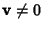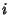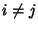## Positive Definite Matrix

A Matrixis positive definite if(1)

for all Vectors. All Eigenvalues of a positive definite matrix are Positive (or, equivalently, the Determinants associated with all upper-left Submatrices are Positive).

The Determinant of a positive definite matrix is Positive, but the converse is not necessarily true (i.e., a matrix with a Positive Determinant is not necessarily positive definite).

A Real Symmetric Matrixis positive definite Iff there exists a Real nonsingular Matrixsuch that(2)

ASymmetric Matrix(3)

is positive definite if(4)

for all.

A Hermitian Matrixis positive definite if

1.for all,

2.for,

3. The element of largest modulus must lie on the leading diagonal,

4..